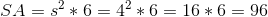## Example Questions

← Previous 1

### Example Question #1 : How To Find The Surface Area Of A Cube

If the surface area of a cube equals 96, what is the length of one side of the cube?

5

3

4

6

4

Explanation:

The surface area of a cube = 6a2 where a is the length of the side of each edge of the cube. Put another way, since all sides of a cube are equal, a is just the lenght of one side of a cube.

We have 96 = 6a→ a2 = 16, so that's the area of one face of the cube.

Solving we get √16, so a = 4

### Example Question #2 : How To Find The Surface Area Of A Cube

The side of a cube has a length of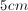. What is the total surface area of the cube?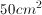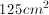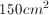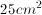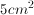Explanation:

A cube has 6 faces. The area of each face is found by squaring the length of the side.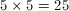Multiply the area of one face by the number of faces to get the total surface area of the cube.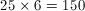### Example Question #1 : How To Find The Surface Area Of A Cube

What is the surface area of a cube if its height is 3 cm?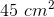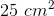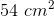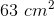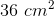Explanation:

The area of one face is given by the length of a side squared.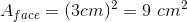The area of 6 faces is then given by six times the area of one face: 54 cm2.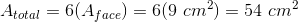### Example Question #1 : How To Find The Surface Area Of A Cube

A sphere with a volume of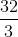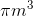is inscribed in a cube, as shown in the diagram below.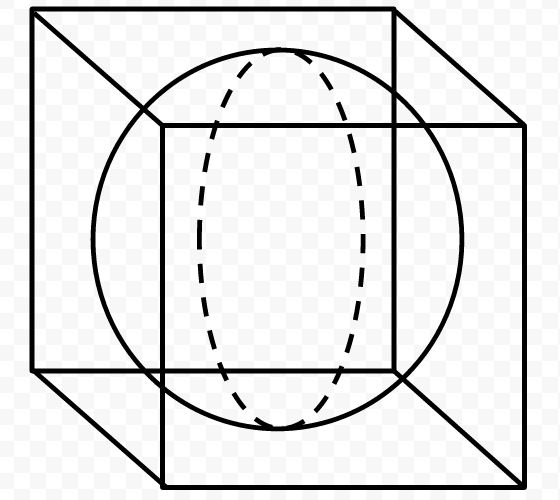What is the surface area of the cube, in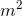?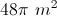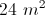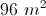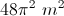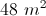Explanation:

We must first find the radius of the sphere in order to solve this problem. Since we already know the volume, we will use the volume formula to do this.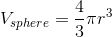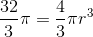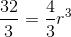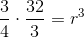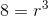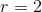With the radius of the sphere in hand, we can now apply it to the cube. The radius of the sphere is half the distance from the top to the bottom of the cube (or half the distance from one side to another). Therefore, the radius represents half of a side length of a square. So in this case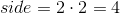The formula for the surface area of a cube is: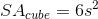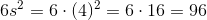The surface area of the cube is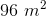### Example Question #5 : How To Find The Surface Area Of A Cube

What is the surface area, in square inches, of a four-inch cube?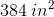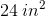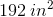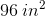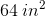Explanation:

To answer this question, we need to find the surface area of a cube.

To do this, we must find the area of one face and multiply it by, because a cube hasfaces that are square in shape and equal in size.

To find the area of a square, you multiply its length by its width. (Note that the length and width of a square are the same.) Therefore, for this data: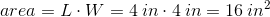We now must multiply the area of one face by 6 to get the total surface are of the cube.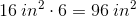Therefore, the surface are of a four-inch cube is.

### Example Question #6 : How To Find The Surface Area Of A Cube

What is the surface area of a cube with a volume of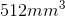? Round your answer to the nearest hundreth if necessary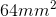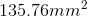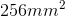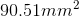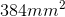Explanation:

First we need to find the side length of the cube. Do that by taking the cube root of the volume.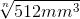=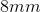Next plug the side length into the formula for the surface area of a cube: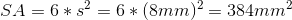### Example Question #7 : How To Find The Surface Area Of A Cube

What is the surface area, in square inches, of a cube with sides measuring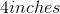?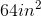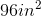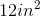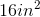Explanation:

The surface area of a cube is a measure of the total area of the surface of all of the sides of that cube.

Since a cube containssquare sides, the surface area istimes the area of a square side.

The area of one square side is sidelengthsidelength, or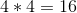in this case. Therefore, the surface area of this cube is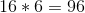square inches.

### Example Question #8 : How To Find The Surface Area Of A Cube

What is the length of the side of a cube whose surface area is equal to its volume?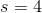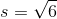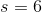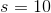There is not enough information to determine the answer.Explanation:

To find the side length of a cube whose surface area is the same as its volume, set the surface area and volume equations of a cube equal to each other, the solve for the side length: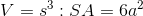Set these two formulas equal to eachother and solve for s.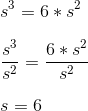### Example Question #9 : How To Find The Surface Area Of A Cube

What is the surface area of a cube with a side of length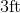?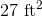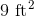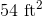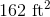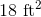Explanation:

To find the surface area of a cube with a given side length,use the formula: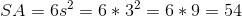### Example Question #10 : How To Find The Surface Area Of A Cube

Find the surface area of a cube whose side length is.To find surface area of a cube, simply calculate the area of one side and multiply it by. Thus,